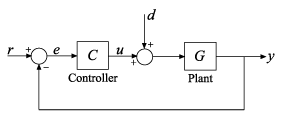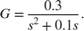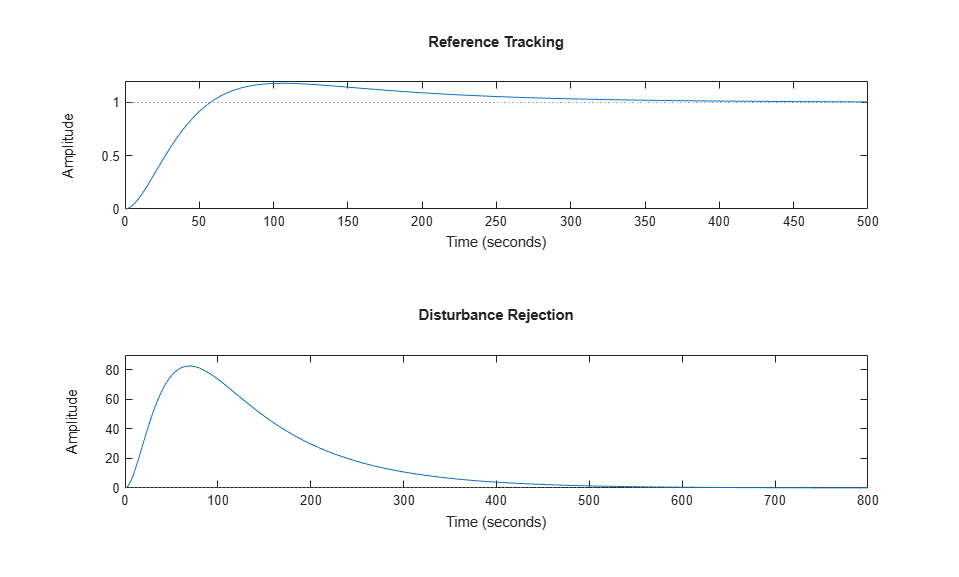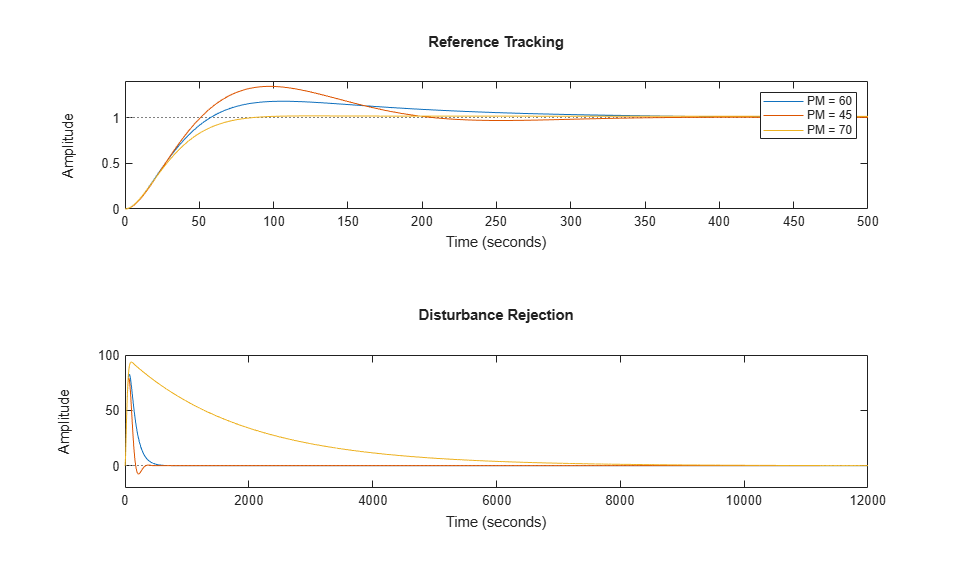# 调节 PID 控制器以侧重参考跟踪或扰动抑制（命令行）G = tf(0.3,[1 0.1 0]); 

wc = 0.03; [C1,info] = pidtune(G,'PI',wc); 

T1 = feedback(G*C1,1); GS1 = feedback(G,C1); subplot(2,1,1); stepplot(T1) title('Reference Tracking') subplot(2,1,2); stepplot(GS1) title('Disturbance Rejection')info.PhaseMargin 
ans = 60.0000 

opts2 = pidtuneOptions('PhaseMargin',45); C2 = pidtune(G,'PI',wc,opts2); T2 = feedback(G*C2,1); GS2 = feedback(G,C2); opts3 = pidtuneOptions('PhaseMargin',70); C3 = pidtune(G,'PI',wc,opts3); T3 = feedback(G*C3,1); GS3 = feedback(G,C3); subplot(2,1,1); stepplot(T1,T2,T3) legend('PM = 60','PM = 45','PM = 70') title('Reference Tracking') subplot(2,1,2); stepplot(GS1,GS2,GS3) title('Disturbance Rejection')DesignFocus 选项对于具有更多可调参数的控制系统更高效。因此，当与 PI 控制器结合使用时，其效果不明显。要查看其效果，请分别使用不同的 DesignFocus 值为相同的带宽和默认相位裕度 (60°) 设计一个 PIDF 控制器。比较结果。

opts4 = pidtuneOptions('DesignFocus','balanced'); % default focus C4 = pidtune(G,'PIDF',wc,opts4); T4 = feedback(G*C4,1); GS4 = feedback(G,C4); opts5 = pidtuneOptions('DesignFocus','disturbance-rejection'); C5 = pidtune(G,'PIDF',wc,opts5); T5 = feedback(G*C5,1); GS5 = feedback(G,C5); opts6 = pidtuneOptions('DesignFocus','reference-tracking'); C6 = pidtune(G,'PIDF',wc,opts6); T6 = feedback(G*C6,1); GS6 = feedback(G,C6); subplot(2,1,1); stepplot(T4,T5,T6) legend('Balanced','Rejection','Tracking') title('Reference Tracking') subplot(2,1,2); stepplot(GS4,GS5,GS6) title('Disturbance Rejection')